Skip to main content

# Achieving low-complexity maximum-likelihood detection for the 3D MIMO code

## Abstract

The 3D Multiple-input multiple-output (MIMO) code is a robust and efficient space-time block code (STBC) for the distributed MIMO broadcasting but suffers from high maximum-likelihood (ML) decoding complexity. In this paper, we first analyze some properties of the 3D MIMO code to show that the 3D MIMO code is fast decodable. It is proven that the ML decoding performance can be achieved with a complexity of O(M4.5) instead of O(M8) in quasi-static channel with M-ary square QAM modulations. Consequently, we propose a simplified ML decoder exploiting the unique properties of the 3D MIMO code. Simulation results show that the proposed simplified ML decoder can achieve much lower processing time latency compared to the classical sphere decoder with Schnorr-Euchner enumeration.

## 1 Introduction

Multiple-input multiple-output (MIMO) is a promising technique that can bring significant improvements to the wireless communication systems. In combination with space-time block code (STBC), it provides higher spectrum efficiency with better communication reliability . In the last decades, MIMO has been widely employed in the latest wireless communication standards such as IEEE 802.11n, 3GPP Long Term Evolution (LTE), WiMAX, and Digital Video Broadcasting-Next Generation Handheld (DVB-NGH). It is also seen as the key technology for the future digital TV terrestrial broadcasting standards .

A so-called space-time-space (3D) MIMO code  was proposed for future TV broadcasting systems, in which the services are delivered by the MIMO transmission in a single-frequency network (SFN). Specifically, it is proposed for a distributed MIMO broadcasting scenario, where TV programs are transmitted by two geographically separated transmission sites, each site equipping two transmit antennas. On the other hand, each receiver has two receive antennas, forming a 4×2 MIMO transmission. The 3D MIMO code has been shown to be robust and efficient in distributed MIMO broadcasting scenarios where there exist strong received signal power imbalances . Hence, it is a promising candidate for MIMO profile of future broadcasting standards. However, the 3D MIMO code suffers from a high computational complexity when the maximum-likelihood (ML) decoding is adopted. The decoding complexity is as high as O(M8) when M-QAM constellation is used. Up to now, no study on the decoding complexity reduction for the 3D MIMO code has been carried out in the literature.

Recently, a lot of efforts have been made in the STBC design to obtain both high code rate and low decoding complexity . The decoding complexity reduction is commonly achieved by exploiting the orthogonality embedded in the STBC codeword. When there exist groupwise orthogonality in the codeword, the joint detection of many information symbols is converted into independent, groupwise detections [6, 10], yielding low decoding complexity. For other cases such as DjABBA code , Biglieri-Hong-Viterbo (BHV) code , Srinath-Rajan code , and Ismail-Fiorina-Sari (IFS) code  in which the orthogonality only exists in a part of information symbols, some symbols can be detected in a groupwise manner once we condition them with respect to other symbols. Such kind of STBCs are referred to as fast decodable STBCs because they achieve ML decoding performance with a reduced order of complexity. However, most of the fast decodable STBCs are not optimized for distributed MIMO broadcasting scenarios, and they are not robust under received signal power imbalance conditions .

A partial interference cancellation (PIC) group decoding scheme has been presented, aiming at reducing the decoding complexity of the STBCs containing groupwise orthogonalities in the codewords [13, 14]. A number of STBCs that are optimized for this decoding scheme have also been proposed [14, 15]. This scheme actually uses a linear equalization to convert the joint detection of a large number of symbols to several groups of ML decodings for few symbols. However, the overall performance of this decoding scheme cannot achieve the ML optimality.

Some alternatives with reduced decoding complexity have been presented for the distributed MIMO broadcasting. Polonen and Koivunen described a STBC with less decoding complexity based on orthogonal basis . However, such a code does not achieve full diversity or full rate for 4×2 MIMO transmissions and therefore performs worse than the 3D MIMO code. A ‘punctured version’ of the 3D MIMO code that possesses full rate with low decoding complexity has also been proposed . However, it does not achieve full diversity and is hence less robust in harsh channel conditions.

In this paper, we propose a reduced-complexity ML decoder for the 3D MIMO code which exploits the embedded orthogonality in the codeword. The main contributions are as follows:

• We propose to modify the original 3D MIMO codeword through some permutations of information symbols which lead to an ML decoding algorithm with reduced complexity without affecting all desirable properties of the 3D MIMO code.

• We prove that the 3D MIMO code is fast decodable. Moreover, we show that the worst-case decoding complexity is O(M4.5) for M-ary square QAM modulations which is the least among all square full-rate STBCs for 4×2 MIMO transmission.

• Based on the unique properties of the new form of 3D MIMO codeword, we propose a novel implementation of the simplified decoder that achieves a lower average complexity in terms of time latency without losing the ML optimality. The proposed implementation is also applicable for other fast decodable STBCs.

The remainder of the paper is organized as follows. Some fundamentals of the MIMO detection are presented in Section 2. In Section 3, the 3D MIMO code is first recalled. Consequently, a modification of the codeword is proposed to facilitate the decoding process. Three important properties of the new codeword are also revealed. With this knowledge, in Section 4, the ML decoder with a worst-case decoding complexity of O(M4.5) is derived. Then, in Section 5, a new implementation of the reduced-complexity ML decoder is described. Section 6 presents the symbol error and complexity performance of the new decoder. Conclusions are drawn in Section 7.

### 1.1 Notations

Vectors and matrices are written in boldface letters. Superscript XT represents transposition of matrix X. xR and xI denote the real and imaginary parts of a complex number x, respectively. The operator $( · ̌ )$ performs the complex real conversion from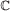to $R 2 × 2$:

$x ̌ ≜ x R − x I x I x R .$
(1)

When the $( · ̌ )$ operator is applied to a matrix $X∈ C m × n$, the operation in (1) is performed for all elements xj,k in the matrix, i.e., the (j,k)th 2×2 submatrix of $X ̌$ is $x ̌ j , k$. For a complex vector $x= [ x 1 , x 2 , … , x n ] T ∈ C n$, the operator $( · ~ )$ separates the real and imaginary parts of the given vector, i.e., $x ~ ≜ [ x 1 R , x 1 I , … , x n R , x n I ] T$. For a matrix X=[x1,x2,…,x n ], where x j is the j th column of X, the operator vec(X) stacks the columns of X to form one column vector, i.e., $vec(X)≜ [ x 1 T , x 2 T , … , x n T ] T$. $vec ( X ) ~$ denotes vectorizing matrix X followed by the real/imaginary part separation. The inner product of two real-valued vectors x and y is denoted by 〈x,y〉=xTy. The n×n identity matrix is denoted by I n . The operator denotes the Kronecker product. Finally, i represents $− 1$.

## 2 System model

### 2.1 MIMO system model

We consider a MIMO transmission with Nt transmit and Nr receive antennas over flat fading channel. The received signal $Y∈ C N r × T$ is

$Y=HX+W,$
(2)

where $X∈ C N t × T$ is the STBC codeword matrix which is transmitted over T channel uses, $W∈ C N r × T$ is a complex-valued additive white Gaussian noise (AWGN) component, $H∈ C N r × N t$ is the channel matrix whose (j,k)th element hj,k denotes the channel coefficient of the link between the k th transmit antenna and the j th receive antenna. The channel is assumed to be quasi-static. That is, the channel coefficients keep constant over the duration of one STBC codeword, but change from one codeword to another. Moreover, hj,k’s are assumed to be independent from each other.

For linear STBCs, the codeword matrix X can be obtained through a linear operation :

$vec ( X ) ~ =G s ~ ,$
(3)

where s=[s1,s2,…,s κ ]T is the vector containing κ independent information symbols. The code rate of STBC is κ/T information symbols per channel use. The generator matrix $G∈ R 2 N t T × 2 κ$ is obtained:

$G≜[ vec ( A 1 ) ~ , vec ( ℬ 1 ) ~ ,…, vec ( ℬ κ ) ~ ],$
(4)

where $A j ∈ C N t × T$ and $ℬ j ∈ C N t × T$ are the complex weight matrices representing the contribution of the real and imaginary parts of the j th information symbol s j in the final codeword matrix.

Separating the real and imaginary parts of the transmitted and received signals and stacking the columns of the codeword, the received MIMO signal (2) can be expressed in an equivalent real-valued form:

$y ~ = H eq s ~ + w ~ ,$
(5)

where $y ~ = vec ( Y ) ~$ and $w ~ = vec ( W ) ~$, and $H eq ∈ R 2 N r T × 2 κ$ is the equivalent channel matrix and is obtained by

$H eq =( I T ⊗ H ̌ )G.$
(6)

Note that the real-valued expression of the signal can be obtained from the complex-valued form via a linear transform. Hence, we will jointly use both real- and complex-valued forms in the sequel.

### 2.2 ML decoding of MIMO signals

Once the channel Heq is known by the receivera, the information symbols can be retrieved from the received signal $y ~$ in (5). The ML solution of the transmitted signal is the combination of information symbols $s ~ =[ s 1 , s 2 ,…, s κ ]$ that minimizes the Euclidian distance between the channel distorted information signal $H eq s ~$ and received signal $y ~$, namely

$s ̂ ML =arg min s ∈ Θ κ ∥ y ~ − H eq s ~ ∥ 2 ,$
(7)

where Θ is the set of the constellation symbols. (7) indicates that the ML solution is found by jointly determining κ independent information symbols. In other words, when the modulation of these symbols is M-QAM, the ML decoding should exhaustively check all Mκ combinations. The search complexity grows dramatically with higher modulation order or larger number of information symbols in one codeword. Hence, the ML decoding is computationally demanding.

### 2.3 Fast ML decoding of MIMO signals

More efficient STBC decoding is achieved with the help of orthogonal-triangular (QR) decomposition [7, 18]. The QR decomposition of the equivalent channel matrix Heq yields Heq=Q R, where $Q∈ R 2 N r T × 2 κ$ is a unitary matrix, and $R∈ R 2 κ × 2 κ$ is an upper triangular matrix. The detailed definitions of the elements in Q and R can be found in the Appendices. Instead of solving (7), the ML solution can be alternatively found by

$s ̂ ML =arg min s ∈ Θ κ ∩ S ∥ z ~ −R s ~ ∥ 2 ,$
(8)

where $z ~ = Q T y ~$ is a linear transformation of received signal and $z ~ ∈ R 2 κ$;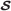is a hypersphere centered on the received signal. Only the codewords inside the hypersphere are checked during the search in order to reduce the search complexity. The size of the hypersphere is represented by its radius. The decoding process is turned into a bounded search over a κ-level tree with complex-valued nodes. Hence, the worst-case decoding complexity is O(Mκ).

Moreover, according to the property of the QR decomposition, some information symbols can be decoded independently from the others if some elements of R are equal to zero. It suggests that the joint search in a high dimension is converted into a bunch of parallel, independent searches in low dimensions. This results in a significant reduction of the worst-case decoding complexity [7, 8, 19].

## 3 3D MIMO code

In this section, we propose a new 3D MIMO codeword that enables low sphere decoding complexity via exchanging the positions of information symbols in the original 3D MIMO codeword. The basic idea behind this modification comes from the facts that the orthogonality embedded in the information symbols essentially enables independent detections and the sphere decoding complexity is mainly determined by the orthogonality among the first several symbols. Hence, exploiting the underlying orthogonality in the codeword and carefully choosing the sequence of information symbols can bring benefits in terms of decoding complexity.

### 3.1 A new proposal of the 3D MIMO codeword

The initially proposed codeword matrix of the 3D MIMO code is explicitly written as

$X 3 D = X golden , 1 − X golden , 2 ∗ X golden , 2 X golden , 1 ∗ = 1 5 α ( s 1 + θ s 2 ) α ( s 3 + θ s 4 ) − α ∗ ( s 5 ∗ + θ s 6 ∗ ) − α ∗ ( s 7 ∗ + θ s 8 ∗ ) i α ̄ ( s 3 + θ ̄ s 4 ) α ̄ ( s 1 + θ ̄ s 2 ) i α ̄ ∗ ( s 7 ∗ + θ ̄ s 8 ∗ ) − α ̄ ∗ ( s 5 ∗ + θ ̄ s 6 ∗ ) α ( s 5 + θ s 6 ) α ( s 7 + θ s 8 ) α ∗ ( s 1 ∗ + θ s 2 ∗ ) α ∗ ( s 3 ∗ + θ s 4 ∗ ) i α ̄ ( s 7 + θ ̄ s 8 ) α ̄ ( s 5 + θ ̄ s 6 ) − i α ̄ ∗ ( s 3 ∗ + θ ̄ s 4 ∗ ) α ̄ ∗ ( s 1 ∗ + θ ̄ s 2 ∗ ) ,$
(9)

where $θ= 1 + 5 2$, $θ ̄ =1−θ$, α=1+i(1−θ), and $α ̄ =1+i(1− θ ̄ )$. It is constructed in a hierarchical manner: eight information symbols (κ=8) are first encoded to two golden codewords , i.e. Xgolden,1 and Xgolden,2, which are consequently arranged in an Alamouti manner  over four channel uses (T=4)b. This results in a code rate of 2 which is full rate for the 4×2 MIMO transmission. Previous study shows that the 3D MIMO code achieves efficient and robust performance. However, since eight information symbols are stacked in one codeword, the ML decoding complexity is up to O(M8).

It was shown that it is possible to achieve lower sphere decoding complexity through permuting the sequence of information symbols . We propose to slightly modify the codeword by exchanging the positions of information symbols (s3,s4) and (s5,s6), yielding a new form of codeword:

$X 3 D , new = 1 5 α ( s 1 + θ s 2 ) α ( s 5 + θ s 6 ) − α ∗ ( s 3 ∗ + θ s 4 ∗ ) − α ∗ ( s 7 ∗ + θ s 8 ∗ ) i α ̄ ( s 5 + θ ̄ s 6 ) α ̄ ( s 1 + θ ̄ s 2 ) i α ̄ ∗ ( s 7 ∗ + θ ̄ s 8 ∗ ) − α ̄ ∗ ( s 3 ∗ + θ ̄ s 4 ∗ ) α ( s 3 + θ s 4 ) α ( s 7 + θ s 8 ) α ∗ ( s 1 ∗ + θ s 2 ∗ ) α ∗ ( s 5 ∗ + θ s 6 ∗ ) i α ̄ ( s 7 + θ ̄ s 8 ) α ̄ ( s 3 + θ ̄ s 4 ) − i α ̄ ∗ ( s 5 ∗ + θ ̄ s 6 ∗ ) α ̄ ∗ ( s 1 ∗ + θ ̄ s 2 ∗ ) .$
(10)

Since we only change the sequence of the information symbols in the codeword (the third and fourth information symbols become the fifth and sixth, respectively, and vice versa) and the information symbols are independent from each other, the new codeword preserves all the good attributes of the original 3D MIMO code in distributed MIMO scenarios. More importantly, this modification is based on the embedded orthogonalities in the 3D MIMO codeword and yields an interesting codeword structure which will be exploited to achieve lower decoding complexity. The advantages brought by the new codeword structure will be highlighted in the following sections.

### 3.2 Key properties of the proposed 3D MIMO codeword

Due to the underlying Alamouti and golden structures, the 3D MIMO code has some unique properties which lead to simplified decoding algorithms. For the modified 3D MIMO code (10) over a 4×2 MIMO channel, the R matrix in (8) is a 16×16 real-valued matrix. Rewrite R in a block-wise form:

$R= R 11 R 12 R 13 R 14 0 R 22 R 23 R 24 0 0 R 33 R 34 0 0 0 R 44 ,$
(11)

where R jk ’s are 4×4 submatrices containing 〈q m ,h n 〉’s with m=4(j−1)+1,…,4j and n=4(k−1)+1,…,4k.

Based on the new codeword in (10) and taking into account (6), (3), and (4), we obtain a few interesting properties of R that can be made use of to achieve a low decoding complexity.

#### Theorem

Theorem 1.R11 is an upper triangular matrix withq1,h2〉=〈q1,h4〉=〈q2,h3〉=〈q3,h4〉=0. □

#### Theorem

Theorem 2.R12 is a null matrix when the channel is quasi-static, i.e.,q j ,h k 〉=0, j=1,2,3,4, and k=5,6,7,8. □

#### Corollary

Corollary 1.R22 is an upper triangular matrix with similar structure as R11, i.e., 〈q5,h6〉=〈q5,h8〉=〈q6,h7〉=〈q7,h8〉=0. □

The proofs of Theorem 1, Theorem 2, and Corollary 1 are presented in the Appendices. The above properties are visualized in Figure 1.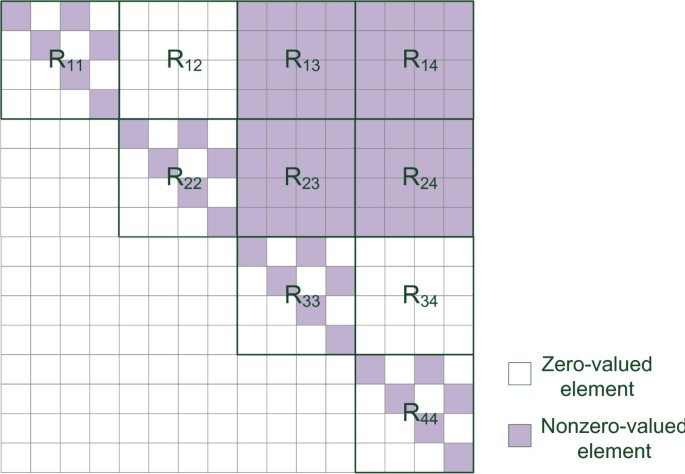Figure 1

#### Remark

Remark 1. Theorem 1 and Corollary 1 actually suggest the independency between real and imaginary parts of the information symbols. For instance, 〈q1,h2〉=〈q1,h4〉=〈q3,h4〉=0 means that the real parts of the first and second received symbols, namely $z ~ (1)$ and $z ~ (3)$, do not contain any contribution from $s 1 I$ and $s 2 I$. Similarly, 〈q2,h3〉=0 means that their imaginary parts, namely $z ~ (2)$ and $z ~ (4)$, do not contain any contribution from $s 1 R$ and $s 2 R$, either. As we will show later, this real/imaginary independency leads to independent and parallel detections for real part and imaginary part, respectively.

The real/imaginary part independency comes from the underlying golden and Alamouti structures. It has been revealed that the complex-valued R matrix of the golden code has a real upper left submatrix , which coincides with the structure as presented in Theorem 1. It shows the real/imaginary part independency of the golden code in its 2×2 codeword matrix. The Alamouti-like arrangement of the two golden codewords, on the other hand, helps create this independency in the 4×4 codeword matrix of the 3D MIMO code.

#### Remark

Remark 2. Theorem 2 indicates that some parts of the information symbols are uncorrelated with others in the received symbols. More precisely, the first two received complex symbols, or equivalently $z ~ (1)$, $z ~ (2)$, $z ~ (3)$, and $z ~ (4)$, do not contain any contribution from information symbols s3 and s4. Hence, a group of six information symbols s1, s2, s5, s6, s7, and s8 can be jointly determined, regardless of the values of s3 and s4. It means that the ML decoding can be achieved by joint searches over six, instead of eight, information symbols. In other words, the ML decoding complexity is expected to be O(M6) instead of O(M8). Therefore, the 3D MIMO code is fast decodable.

It should be noted that Theorem 2 is partially enabled by the embedded Alamouti structure in the codeword. The channel coefficients should be constant within the duration of one codeword to validate the orthogonalities in the Alamouti structure. Hence, Theorem 2 is only valid in the quasi-static channelsc.

### 3.3 Comparison with the original 3D MIMO codeword

Figure 1 illustrates the R matrix of the new 3D MIMO codeword. Compared with the original one as shown in Figure 2, the new structure is actually more favorable for the MIMO decoding. In the new codeword, the contributions of information symbol groups (s1,s2) and (s3,s4) are totally uncorrelated in the received signal, which means that the ML detection of eight information symbols can be achieved by two independent and less complex detections of six information symbols. Moreover, the structures of R11 and R22 enable the independent detections of real and imaginary parts of (s1,s2) and (s3,s4), which leads to further complexity reduction. Yet, this real/imaginary part separation is not straightforward in the original codeword.Figure 2

It should be emphasized that the new codeword only changes the sequence of the information symbols in the codeword to facilitate the decoding process. It does not affect all the good properties of the 3D MIMO code.

## 4 Proposed ML decoder with low complexity

In this section, a low-complexity ML decoding algorithm exploiting the unique properties highlighted in the previous section is proposed for the 3D MIMO code. Generally speaking, the complexity reduction is achieved in two steps. Based on Theorem 2, the joint detection of eight information symbols is converted into two partially independent detections of six information symbols. This step reduces the worst-case decoding complexity from O(M8) to O(M6). Consequently, using Theorem 1 and Corollary 1, the detections of complex information symbols are converted into independent detections of real and imaginary parts, which further reduces the worst-case complexity to O(M4.5).

### 4.1 Groupwise parallel detections

We divide the information symbols and received symbols into four groups, i.e., $a= [ s 1 , s 2 ] T ~$, $b= [ s 3 , s 4 ] T ~$, $c= [ s 5 , s 6 ] T ~$, $d= [ s 7 , s 8 ] T ~$, $z 12 = [ z 1 , z 2 ] T ~$, $z 34 = [ z 3 , z 4 ] T ~$, $z 56 = [ z 5 , z 6 ] T ~$, and $z 78 = [ z 7 , z 8 ] T ~$. Taking into account the structure of R and Theorem 2, the decoding metric in (8) can be rewritten as

$∥ z ~ − R s ~ ∥ 2 = ∥ z 12 − R 11 a − R 13 c − R 14 d ∥ 2$
(12)
$+ ∥ z 34 − R 22 b − R 23 c − R 24 d ∥ 2 + ∥ z 56 − R 33 c − R 34 d ∥ 2 + ∥ z 78 − R 44 d ∥ 2 .$
(13)

From (12) and (13), it can be seen that the contributions from the information symbol groups a and b are uncorrelated in the received symbol. For instance, z12 does not contain any information from b, and z34 is irrelevant to a, either. This enables us to use groupwise conditional detections to retrieve the ML solutions .

In particular, the ML solution $s ̂ ML = [ a ̂ , b ̂ , c ̂ , d ̂ ] T$ is achieved in two search steps, namely, a joint ‘outer’ search for $[ c ̂ , d ̂ ]$:

(14)

and two independent ‘inner’ searches for $a ̂$ and $b ̂$, respectively:

$a ̂ = a ( ∗ ) ( c ̂ , d ̂ ) , b ̂ = b ( ∗ ) ( c ̂ , d ̂ ) ,$
(15)

where

$a ( ∗ ) ( c , d ) = arg min a ∈ Θ 2 ∥ v 12 − R 11 a ∥ 2 ,$
(16)
$b ( ∗ ) ( c , d ) = arg min b ∈ Θ 2 ∥ v 34 − R 22 b ∥ 2 ,$
(17)

with v12=z12R13cR14d, v34=z34R23cR24d. The outer search is carried out over the combinations of [c,d]. For a given [c,d], the search of a and the search of b are performed in parallel. The concatenation of the outer and inner searches (either a or b) results in a joint search of six information symbols. Therefore, the worst-case decoding complexity is reduced from O(M8) to O(M6). We note that this complexity reduction does not rely on the constellation that is adopted by the information symbols. In other words, the 3D MIMO code requires a worst decoding complexity of O(M6) for arbitrary modulation.

### 4.2 Independent detections of real and imaginary parts

If square-shape QAM modulations are considered, the decoding complexity can be further improved. The square M-QAM symbol can be separated into two independent $M −PAM$ symbols on the real and imaginary axes, respectively. Using Theorem 1 and Corollary 1, the real and imaginary parts can be decoded separately. Take the detection of a as an example. Denote its real and imaginary parts as $a R = [ s 1 R , s 2 R ] T$ and $a I = [ s 1 I , s 2 I ] T$, respectively. Given [c,d] and using Theorem 1, the detection of a in (16) is rewritten as 

$a ̂ R = arg min a R ∈ Ψ 2 ∥ v 12 R − R 11 R a R ∥ 2 , a ̂ I = arg min a I ∈ Ψ 2 ∥ v 12 I − R 11 I a I ∥ 2 ,$
(18)

where Ψ is the set of $M −PAM$ constellation symbols. $v 12 R = [ v 1 R , v 2 R ] T$, and $v 12 I = [ v 1 I , v 2 I ] T$; $R 11 R$ and $R 11 I$ are tailored upper triangular matrices associated with real and imaginary parts, respectively:

$R 11 R = R 11 ( 1 , 1 ) R 11 ( 1 , 3 ) 0 R 11 ( 3 , 3 ) , R 11 I = R 11 ( 2 , 2 ) R 11 ( 2 , 4 ) 0 R 11 ( 4 , 4 ) .$
(19)

(18) means that the detections of real and imaginary parts are similar and can be performed separately. Take the real part as an example. We apply again the conditional detection here. For a given $s 2 R$, the metric for the real part detection becomes

$∥ v 12 R − R 11 R a R ∥ 2 = w 1 R − R 11 ( 1 , 1 ) s 1 R 2 + ( w 2 R ) 2 ,$
(20)

where $w 1 R = v 1 R − R 11 (1,3) s 2 R$ and $w 2 R = v 2 R − R 11 (3,3) s 2 R$. For a given $s 2 R$, the best $s 1 R$ that minimizes the decoding metric can alternatively be found by minimizing a quadratic function of $s 1 R$ given on the right-hand side of (20). The best solution of $s 1 R$ is easily found by

$s ¯ 1 R =Q v 1 R − R 11 ( 1 , 3 ) s 2 R R 11 ( 1 , 1 ) ,$
(21)

where Q(·) is the slicing operation providing the PAM symbol that is closest to the given value. The best combination of $[ ŝ 1 R , ŝ 2 R ] T$ given [c,d] is obtained after testing (21) with all ($M$) possible values of $s 2 R$:

(22)

Consequently, $ŝ 1 R$ is obtained by using the solution $ŝ 2 R$ in (21). Similar process can be applied to solve the imaginary parts. The best solution of $[ ŝ 1 I , ŝ 2 I ] T$ given [c,d] can be found by

(23)

where

$s ¯ 1 I =Q v 1 I − R 11 ( 2 , 4 ) s 2 I R 11 ( 2 , 2 ) .$
(24)

$ŝ 1 I$ is computed by applying the solution $ŝ 2 R$ in (24).

Using the same technique, the best solutions of b in (17) can also be converted into independent detections of bR and bI. Substituting R11, v1, v2, s1, and s2 in (21), (22), (23), and (24) by R22, v3, v4, s3, and s4, respectively, it yields the detections for s3 and s4. In general, for a given [c,d], the search of two complex symbols [a,b] is turned into four independent searches of $M$ PAM symbols. The resulting overall complexity to decode a whole codeword is O(M4.5).

In summary, the 3D MIMO code requires a worst decoding complexity of O(M6) for any modulation scheme and O(M4.5) for square M-QAM modulations. The comparisons with other state-of-the-art fast decodable STBCs that are full rate for 4×2 MIMO transmissions are presented in Table 1. It can be seen that the 3D MIMO code is among the simplest full-rate STBCs when the square QAM modulations are considered.

## 5 Proposed implementation of the simplified ML decoder

In the previous sections, we have illustrated the fast decodability of the 3D MIMO code in theory. With this knowledge, we propose an implementation of the simplified ML decoder that can be used in practice. Using the two-stage tree search structure and leveraging the symmetry structure in the codeword, the proposed implementation requires a low average complexity in practice. Moreover, various performance complexity trade-offs can be easily achieved by replacing the sphere decoder by other suboptimal tree search algorithms such as K-best algorithm  and fixed-complexity sphere decoder .

### 5.1 Two-stage decoding structure

Recall that the fast decodability is achieved by concatenating the joint search of four complex symbols and several detections in parallel. Figure 3 presents the general structure of the proposed simplified ML decoder. A detailed pseudocode is presented in Algorithms 1, 2, 3, and 4 so that the proposed decoder can be implemented without major effort.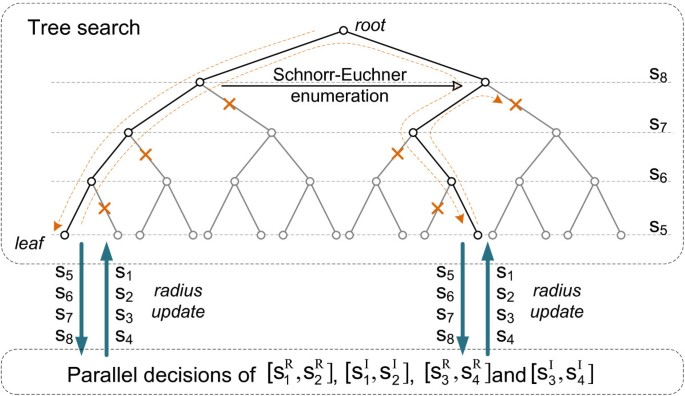Figure 3

#### Algorithm 1 Simple ML decoder for 3D MIMO code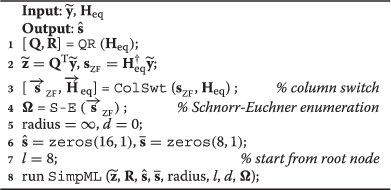#### Algorithm 2 Simple ML decoder SimpML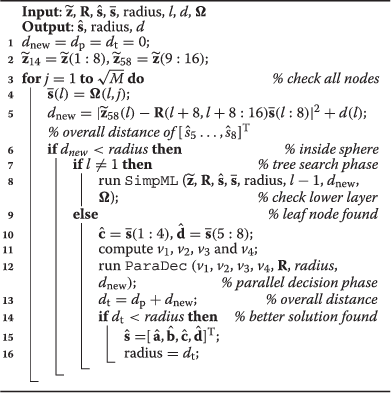#### Algorithm 3 Parallel decision algorithm ParaDec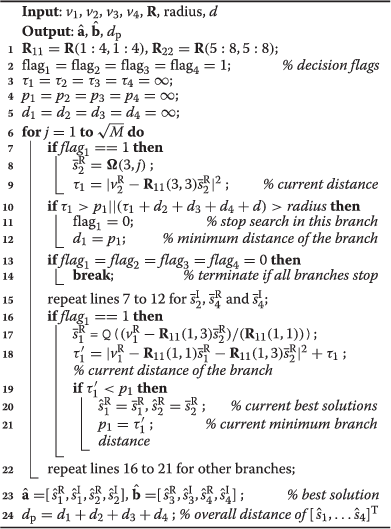#### Algorithm 4 Column switch algorithm ColSwt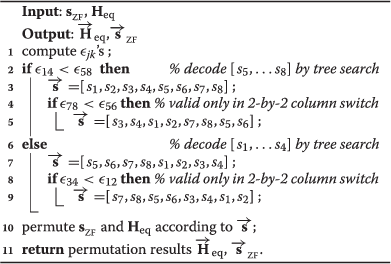#### 5.1.1 Four-level tree search phase

The joint detection of [c,d] is realized by a complex sphere decoder with Schnorr-Euchner enumeration, which is visualized by the search over a four-level tree as shown in Figure 3. The nodes of the same level represent all the solutions of a complex information symbol. Each path from the root to a leaf node represents a possible combination of [c,d].

The details of the tree search is explicitly presented in Algorithm 2. The search starts from the root node and traverses the nodes of lower levels in a depth-first manner. An adaptive search radius is used to speed up the convergence of the algorithm by limiting the search within a hypersphere. For the node under checking, the partial distance resulted by the current path is compared with the radius. If the partial distance is smaller than the radius, the search moves on to the children nodes on the next level. Otherwise, the search jumps to another sibling node on the current level. When all the nodes of the level have already been checked, the search goes back to the upper level. The radius is initially set to infinity and is adaptively decreased according to the best solution already found in the search. Specifically, the radius is updated, taking into account the best combination of [c,d], and [a,b] (line 16 of Algorithm 2). The latter is obtained from the parallel decisions phase. The tree search is terminated when all the nodes within the hypersphere have been checked. The best solution is the ML solution.

The sequence in which the sibling nodes are visited is determined according to the their partial distances in an ascending order. This is to guarantee that the promising candidates are visited first in order to reduce the search complexity. This ordering process is referred to as the Schnorr-Euchner enumeration [18, 27, 28]. It can simply be implemented by a lookup table [29, 30] (line 4 in Algorithm 1), and its complexity is merely the computation of the linear estimation $ŝ ZF$.

#### 5.1.2 Parallel decision phase

Once a leaf node is achieved in the tree search, a better solution of [c,d] is found. Consequently, the tree search process is suspended, and the new [c,d] is used to trigger the parallel detections of the rest symbols.

The parallel detection is depicted in Figure 4. The implementation details are presented in Algorithm 3. As shown in Figure 4, the detections of $[ s 1 R , s 2 R ]$, $[ s 1 I , s 2 I ]$, $[ s 3 R , s 4 R ]$, and $[ s 3 I , s 4 I ]$ are carried out in parallel. For each branch, a one-level sphere decoder is used to traverse all possible PAM symbols as given in (22). The visiting sequence is also determined by the Schnorr-Euchner enumeration. The detections in the different branches are synchronized by a common clock signal because the operations are exactly the same for all branches. All branches simultaneously check the first candidate PAM symbol and then move on to the second one, and so on.Figure 4

Moreover, we propose a mechanism that terminates the search in each branch not only based on its own results but also taking into account the results from the other branches. In particular, once the best solution of the j th branch is found ahead of the others, the resulting branch distance d j is recorded and shared with other branches to speed up the overall search process.

Take the search of the first branch as an example. The most promising PAM symbol in the unchecked symbol list is assigned to $s ¯ 2 R$ (line 8 in Algorithm 3). The partial distance τ1 is calculated (line 9 in Algorithm 3). The search is terminated in two cases: (a) if this partial distance is greater than the current minimum branch distance (τ1>p1) and (b) if the overall distance is beyond the current radius of the sphere decoder in the tree search phase ((τ1+d2+d3+d4+d)>radius).

Once the searches on all the branches are terminated, the solution [a,b] and the resulting distance dp are returned to the tree search phase. The tree search process is resumed. The overall distance is compared with the current radius (line 14 in Algorithm 2) to determine whether the current solution is a better one. If a better solution is found, the radius is updated accordingly (line 16 in Algorithm 2). The tree search process is moved on to the next unchecked node.

### 5.2 Column switch based on ZF estimation

In the proposed algorithm, the search of eight symbols is divided into a tree search for four symbols and parallel detections for the other four symbols. Due to the symmetric structure of the codeword matrix (10), some parts of the codewords can be exchanged without changing the properties of the 3D MIMO code. For instance, we have the same properties as illustrated in Section 3 after exchanging the positions of [s1,s2,s3,s4] with [s5,s6,s7,s8]. Similarly, if we exchange [s1,s2] with [s3,s4] and exchange [s5,s6] with [s7,s8] simultaneously, the structure of R matrix maintains, as well. That is to say, besides the original symbol sequence, the proposed low-complexity decoding algorithm is also valid with other three permuted symbol sequences, i.e., [s5,s6,s7,s8,s1,s2,s3,s4], [s3,s4,s1,s2,s7,s8,s5,s6], and [s7,s8,s5,s6,s3,s4,s1,s2].

The exchanging of the symbol sequences can be achieved by permuting the corresponding columns in the equivalent channel matrix Heq. Note that the aforementioned column permutations do not affect the decoding performance. This permits us to choose the symbols that will be determined by the tree search and the ones that will be decoded in the parallel detections.

The proposed column switch method is presented in Algorithm 4. The basic idea is to use the tree search to determine the more difficult half part and use the parallel detections to find the easier half part. The reason behind this idea is that the parallel decoding is more efficient to decode the reliable symbols separately. The more accurate the linear estimation, the faster is the convergence speed for each individual detection branch. On the other hand, the tree search phase is a joint serial detection in nature which is more suitable to decode those unreliable symbols.

The next question is how to properly choose the unreliable symbols. In the literature, Barbero and Thompson proposed to sort the decoding sequence based on the norm of subchannels in the fixed-complexity sphere decoder . However, it is not applicable here because the 3D MIMO code achieves full diversity, and the equivalent subchannels have similar norm values. In addition, as we have to maintain the structure of the R matrix, the unconstrained subchannel sorting proposed in  is not applicable, either.

Alternatively, we propose to sort the information symbols according to the aggregate error of the linear estimation:

$ε jk = ∑ l = j k | s ̂ ZF ( l ) − s ZF ( l ) | 2 ,$
(25)

where $s ZF = H eq ‡ y$ is the unconstrained estimation of the information symbols in which $H eq ‡$ represents the inverse of the equivalent channel matrix; $s ̂ ZF =Q( s ZF )$ is the constellation point that is closest to sZF. The metric is the distance between the estimated information symbols and the nearest constellation points, i.e., an indicator of the estimation accuracy.

Using (25), the decoding sequence can be determined in two levels. We first compare the aggregate errors of the first half and second half parts of the symbols (line 2 in Algorithm 4). The half with worse accuracy is assigned to the tree search (put in the latter part of the decoding sequence). Consequently, within this half part, the errors of the first two symbols and the second two are compared. The two symbols with worse accuracy are put closer to the root of the tree. If this two-symbol-by-two-symbol exchange takes place in the second half of the symbols which are to be decoded using the tree search, the same two-symbol-by-two-symbol exchange should be done accordingly in the other half in order to maintain the structure of the R matrix. If only the symbol exchange between the two halves of the symbols is carried out, it is referred to as ‘4-by-4 column switch’. Otherwise, if the exchange within each half is also performed, it is called ‘2-by-2 column switch’. The advantage of the column switch will be shown in the next section.

## 6 Simulation results

### 6.1 BER performance

Figure 5 presents the uncoded symbol error rate of the proposed simplified decoders in quasi-static independent Rayleigh flat fading channel. The performances of the ML decoder and the sphere decoder with Schnorr-Euchner (S-E) enumeration proposed by Guo and Nilsson  are also given as references. The Guo-Nilsson sphere decoder is a low-complexity implementation of sphere decoder with S-E but is sub-optimal in terms of symbol error rate. It can be seen that the proposed decoders achieve the same performance as ML decoder with both QPSK and 16-QAM modulations. In addition, the proposed decoders outperform the Guo-Nilsson sphere decoder with 16-QAM modulation. A gain of around 0.7 dB can be observed at symbol error rate level of 1×10−4.Figure 5

### 6.2 Computational complexity

Figures 6 and 7 present the complexity in terms of number of visited nodes for decoding each codeword with QPSK and 16-QAM, respectively. For the proposed decoders, this number is calculated as the number of visited nodes in the tree search phase plus the maximum visited nodes among the four search branches. The Guo-Nilsson sphere decoder is also given as a reference. Since the processing of each node requires roughly the same operations for both decoders, these experiments actually give the comparison of the processing time latency [19, 28]. It can be seen from the results that the proposed decoders require much less processing time than the ML decoder which needs to traverse all M8 possibilities. In the QPSK case, the proposed decoders always yield less latency than the Guo-Nilsson sphere decoder. For instance, the proposed decoder with the 2-by-2 column switch visits only 254.6 nodes on an average at SNR of 0 dB. Compared with the Guo-Nilsson decoder which visits 1276.6 nodes at SNR of 0 dB, the proposed one achieves a processing time reduction of 80%. The reductions are 50% and 49% at 10 and 20 dB, respectively. In addition, the improvements brought by the proposed column switch technique can also be seen in the results. For instance, the 2-by-2 column switch yields a processing time reduction of 38% at 0 dB compared with the decoder without column switch. This improvement is less significant in high-SNR region (e.g., greater than 15 dB). In the 16-QAM case (see Figure 7), the proposed decoder with the 2-by-2 column switch also brings processing time reduction in low-SNR region. At 8 dB, it visits 1301.1 nodes on average, yielding a time reduction of 84% compared with the Guo-Nilsson decoder. The proposed decoder needs similar time latency as the Guo-Nilsson decoder in higher-SNR region (e.g., greater than 15 dB). Taking into account the symbol error rate performance given in Figure 5, 815 dB is the SNR range where the error correction ability of the channel coding will be carried out significantly. That is to say, the improvements are achieved in a SNR region of interest.Figure 6Figure 7

Figures 8 and 9 give the overall required multiplications to decode each codeword. For the proposed decoders, the multiplications spent by the tree search and by all the four search branches are taken into account. The computation overheads such as the QR decomposition and the linear estimation are also included in the results to give the overall complexity of the decoders. It can be seen from the results that in the QPSK case, the proposed decoder with the 2-by-2 column switch spends 11%, 21%, and 3% more multiplications than the Guo-Nilsson decoder at a SNR of 0, 6, and 20 dB, respectively. In the 16-QAM case, it needs 5% less multiplication at 8 dB but spends 85% and 9% more multiplications at 14 and 28 dB, respectively. However, it is worth noting that with the cost of increased multiplications, the proposed decoders provide less processing latencies. For instance, the proposed decoder with the 2-by-2 column switch achieves 62% processing time reduction at SNR of 6 dB with QPSK and 42% reduction at 14 dB with 16-QAM, respectively.Figure 8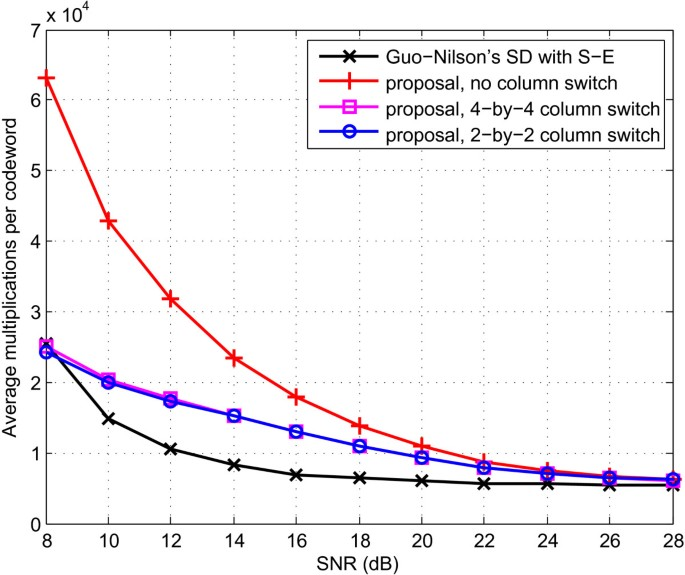Figure 9

Finally, Figures 10 and 11 present the overall divisions spent by the decoders. The proposed decoders require less divisions than the Guo-Nilsson. For instance, the proposed decoder with the 2-by-2 column switch requires 47%, 9%, and 5% less divisions at SNR of 0, 10, and 20 dB, respectively, with QPSK. In the 16-QAM case, it achieves 79% reduction of divisions at 8 dB. In the meantime, the two decoders spend roughly the same number of divisions in higher-SNR region, e.g., greater than 18 dB.Figure 10Figure 11

In general, we can see the different trade-offs achieved by the different decoders. The proposed decoder achieves ML performance with less time latency and less divisions than the Guo-Nilsson. On the other hand, the Guo-Nilsson decoder needs less multiplications with some performance loss with 16-QAM.

## 7 Conclusion

The 3D MIMO code has been shown to be efficient and robust in distributed MIMO scenarios. Yet, it suffers from high ML decoding complexity. In this paper, we first proposed a new form of the the 3D MIMO codeword and investigated some important properties of the new codeword. With these properties, the 3D MIMO code is proven to be fast decodable. Consequently, we proposed a reduced-complexity ML decoder for the 3D MIMO code which offers the same performance as that of the ML decoder. The simulation results demonstrate that the novel low-complexity decoder yields much less processing time latency than the classical Guo-Nilsson sphere decoder with Schnorr-Euchner enumeration. Moreover, the proposed 2-by-2 column switch technique can significantly reduce the average decoding complexity, especially with the 16-QAM modulation.

## Endnotes

a We assume that the receiver has perfect knowledge of the channel in our work. In practice, the channel coefficients should be estimated using some channel estimation techniques.

b Note that this construction is different from those of the quasi-orthogonal code  and the EAST code .

c The fast decodability of the other STBCs such as DjABBA, BHV, Srinath-Rajan, and IFS codes also requires quasi-static channel assumption.

## Appendix

### Definition of the QR decomposition

If we write Heq=[h1,…,h2κ], the Heq’s QR decomposition Heq=Q R is achieved by Gram-Schmidt procedure such that $Q≜[ q 1 ,…, q 2 κ ]$, where the columns q j ’s are orthogonal, and

$R≜ ∥ r 1 ∥ 2 〈 q 1 , h 2 〉 ⋯ 〈 q 1 , h 2 κ 〉 0 ∥ r 2 ∥ 2 ⋯ 〈 q 2 , h 2 κ 〉 ⋮ ⋮ ⋱ ⋮ 0 0 ⋯ ∥ r 2 κ ∥ 2 ,$
(26)

where r1=h1, $r j = h j − ∑ k = 1 j − 1 〈 q k , h j 〉 q k$, q j =r j /r j , j=1,…,2κ.

### Proof of Theorem 1

Based on Heq, after some straightforward computation, it yields 〈h1,h2〉=〈h1,h4〉=〈h2,h3〉=〈h3,h4〉=0. According to the definition of QR decomposition, q1=h1/h1. Hence, 〈q1,h2〉=〈q1,h4〉=0.

In addition, r2=h2−〈q1,h2q1=h2, q2=r2/r2=h2/h2. Taking into account that 〈h2,h3〉=0, it yields 〈q2,h3〉=0.

Moreover, $r 3 = h 3 − ∑ j = 1 2 〈 q j , h 3 〉 q j = h 3 −〈 q 1 , h 3 〉 q 1$ and q3=r3/r3=(h3−〈q1,h3q1)/r3. Therefore, 〈q3,h4〉=(〈h3,h4〉−〈q1,h3〉〈q1,h4〉)/r3=0.

This completes the proof of Theorem 1.

### Proof of Theorem 2

Based on Heq, after some straightforward computation, it yields 〈h j ,h k 〉=0, j=1,2,3,4, and k=5,6,7,8. Using q1=h1/h1 and q2=h2/h2 which have been proven in the proof of Theorem 1, it yields 〈q j ,h k 〉=0, j=1,2, and k=5,6,7,8.

Using q3=(h3−〈q1,h3q1)/r3 which has been proven in the proof of Theorem 1, it yields 〈q3,h k 〉=(〈h3,h k 〉−〈q1,h3〉〈q1,h k 〉)/r3=0, k=5,6,7,8. Similarly, since q4=(h4−〈q2,h4q2)/r4, it yields 〈q4,h k 〉=(〈h4,h k 〉−〈q2,h4〉〈q2,h k 〉)/r4=0, k=5,6,7,8.

This completes the proof of Theorem 2.

### Proof of Corollary 1

Using the similar method as in the proof of Theorem 1, it can be computed from the definition of Heq that 〈h5,h6〉=〈h5,h8〉=〈h6,h7〉=〈h7,h8〉=0. In addition, using Theorem 2, it can be obtained that q5=h5/h5. Hence, 〈q5,h6〉=〈q5,h8〉=0.

Using 〈q5,h6〉=0 and Theorem 2, it yields q6=h6/h6. Hence, 〈q6,h7〉=0.

Finally, using 〈q6,h7〉=0 and Theorem 2, it yields r7=h7−〈q5,h7q5 and q7=r7/r7=(h7−〈q5,h7q5)/r7. Therefore, 〈q7,h8〉=(〈h7,h8〉−〈q5,h7〉〈q5,h8〉)/r7=0.

This completes the proof of Corollary 1.

## References

1. 1.

Tarokh V, Seshadri N, Calderbank A: Space-time codes for high data rate wireless communication: performance criterion and code construction. IEEE Trans. Inf. Theory 1998, 44(2):744-765. 10.1109/18.661517

2. 2.

DVB: TM-MIMO. . Accessed 29 Jan 2014 http://www.dvb.org/groups/TM-MIMO

3. 3.

Nasser Y, Hélard JF, Crussière M: 3D MIMO scheme for broadcasting future digital TV in single-frequency networks. Electron. Lett 2008, 44(13):829-830. 10.1049/el:20080061

4. 4.

Liu M, Crussière M, Hélard M, Hélard JF: Distributed MIMO schemes for the future digital video broadcasting Paper presented at the 20th international conference on telecommunications (ICT). Casablanca, Morocco0; 6–8 May 2013.

5. 5.

Sharma N, Papadias CB: Full-rate full-diversity linear quasi-orthogonal space-time codes for any number of transmit antennas. EURASIP J. Appl. Signal Process 2004, 2004: 1246-1256. 10.1155/S1110865704402339

6. 6.

Dao DN, Yuen C, Tellambura C, Guan YL, Tjhung TT: Four-group decodable space–time block codes. IEEE Trans. Signal Process 2008, 56: 424-430.

7. 7.

Biglieri E, Hong Y, Viterbo E: On fast-decodable space-time block codes. IEEE Trans. Inf. Theory 2009, 55(2):524-530.

8. 8.

Srinath K, Rajan B: Low ML-decoding complexity, large coding gain, full-rate, full-diversity STBCs for 2 ×2 and 4 ×2 MIMO systems. IEEE J. Sel. Topics Signal Process 2009, 3(6):916-927.

9. 9.

Sinnokrot MO, Barry JR, Madisetti VK: Embedded Alamouti space-time codes for high rate and low decoding complexity. In Paper presented at the 42nd Asilomar conference on signals, systems and computers. Pacific Grove, CA, USA; 3–6 Nov 2008:1749-1753.

10. 10.

Ren TP, Guan YL, Yuen C, Shen RJ: Fast-group-decodable space-time block code. Paper presented at the IEEE information theory workshop (ITW). Cairo, Egypt; 6–8 Jan 2010.

11. 11.

Ismail A, Fiorina J, Sari H: A new family of low-complexity STBCs for four transmit antennas. IEEE Trans. Wireless Commun 2013, 12(3):1208-1219.

12. 12.

Hottinen A, Tirkkonen O, Wichman R: Multi-antenna Transceiver Techniques for 3G and Beyond. West Sussex, England: Wiley; 2003.

13. 13.

Dai L, Sfar S, Letaief K: An efficient detector for combined space-time coding and layered processing. IEEE Trans. Commun 2005, 53(9):1438-1442. 10.1109/TCOMM.2005.855016

14. 14.

Guo X, Xia XG: On full diversity space-time block codes with partial interference cancellation group decoding. IEEE Trans. Inf. Theory 2009, 55(10):4366-4385.

15. 15.

Zhang W, Xu T, Xia XG: Two designs of space-time block codes achieving full diversity with partial interference cancellation group decoding. IEEE Trans. Inf. Theory 2012, 58(2):747-764.

16. 16.

Polonen K, Koivunen V: Reduced complexity space-time coding in single-frequency networks. Paper presented at the IEEE wireless communications and networking conference (WCNC). Cancun, Mexico; 28–31 March 2011.

17. 17.

Liu M, Hélard M, Crussière M, Hélard JF: Distributed MIMO coding scheme with low decoding complexity for future mobile TV broadcasting. Electron. Lett 2012, 48(17):1079-1081. 10.1049/el.2012.1778

18. 18.

Agrell E, Eriksson T, Vardy A, Zeger K: Closest point search in lattices. IEEE Trans. Inf. Theory 2002, 48(8):2201-2214. 10.1109/TIT.2002.800499

19. 19.

Sinnokrot M, Barry J: Fast maximum-likelihood decoding of the golden code. IEEE Trans. Wireless Commun 2010, 9: 26-31.

20. 20.

Belfiore J, Rekaya G, Viterbo E: The golden code: a 2 ×2 full-rate space-time code with nonvanishing determinants. IEEE Trans. Inf. Theory 2005, 51(4):1432-1436. 10.1109/TIT.2005.844069

21. 21.

Alamouti S: A simple transmit diversity technique for wireless communications. IEEE J. Sel. Areas Commun 1998, 16(8):1451-1458. 10.1109/49.730453

22. 22.

Jithamithra G, Rajan BS: Minimizing the complexity of fast sphere decoding of STBCs. Paper presented at the IEEE international symposium on information theory (ISIT). St. Petersburg, Russia; 31 Jul–05 Aug 2011.

23. 23.

Sirianunpiboon S, Wu Y, Calderbank A, Howard S: Fast optimal decoding of multiplexed orthogonal designs by conditional optimization. IEEE Trans. Inf. Theory 2010, 56(3):1106-1113.

24. 24.

Oggier F, Rekaya G, Belfiore JC, Viterbo E: Perfect space–time block codes. IEEE Trans. Inf. Theory 2006, 52(9):3885-3902.

25. 25.

Wong K, Tsui C, Cheng R, Mow W: A VLSI architecture of a K-best lattice decoding algorithm for MIMO channels. Paper presented at the IEEE international symposium on circuits and systems (ISCAS). Scottsdale, AZ, USA; 26–29 May 2002.

26. 26.

Barbero L, Thompson J: Fixing the complexity of the sphere decoder for MIMO detection. IEEE Trans. Wireless Commun 2008, 7(6):2131-2142.

27. 27.

Schnorr C, Euchner M: Math Program. 1994, 66: 181-191. 10.1007/BF01581144

28. 28.

Guo Z, Nilsson P: Reduced complexity Schnorr-Euchner decoding algorithms for MIMO systems. IEEE Commun. Lett 2004, 8(5):286-288. 10.1109/LCOMM.2004.827376

29. 29.

Wiesel A, Mestre X, Pages A, Fonollosa J: Efficient implementation of sphere demodulation. Paper presented at the 4th IEEE workshop on signal processing advances in wireless communications (SPAWC). Rome, Italy; 15–18 June 2003.

30. 30.

Tsai P, Chen W, Lin X, Huang M: A 4 ×4 64-QAM reduced-complexity K-best MIMO detector up to 1.5 Gbps. Paper presented at the IEEE international symposium on circuits and systems (ISCAS), Paris, France, 30 May–2 June 2010, pp. 3953–3956

Download references

## Acknowledgements

This work has been supported by French ANR ‘Mobile Multi-Media (M3)’ project and ‘Pôle Images & Réseaux’.

## Author information

Authors

### Corresponding author

Correspondence to Matthieu Crussière.

## Additional information

### Competing interests

The authors declare that they have no competing interests.

## Authors’ original submitted files for images

Below are the links to the authors’ original submitted files for images.

## Rights and permissions

Open Access This article is distributed under the terms of the Creative Commons Attribution 2.0 International License ( https://creativecommons.org/licenses/by/2.0 ), which permits unrestricted use, distribution, and reproduction in any medium, provided the original work is properly cited.

Reprints and Permissions

## About this article

### Cite this article

Liu, M., Crussière, M., Hélard, M. et al. Achieving low-complexity maximum-likelihood detection for the 3D MIMO code. J Wireless Com Network 2014, 20 (2014). https://doi.org/10.1186/1687-1499-2014-20

Download citation

• Received:

• Accepted:

• Published:

### Keywords

• MIMO
• Space-time codes
• Maximum-likelihood decoding
• Computational complexity